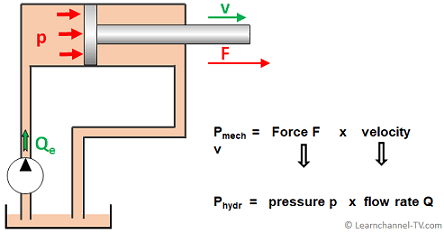# Hydraulic Power

## Hydraulic Power or Fluid Power

The hydraulic power is directly related to the volume flow Q and pressure p. Or in another words: The higher the pressure and the greater the flow rate, the more power you get:

Hydraulic power Phydr  =   Pressure p  X  Flow Rate Q
This equation can be explained most simply using the example of a hydraulic cylinder, which converts hydraulic power into mechanical power:Relation between Hydraulic Power and Mechanical Power

In practice, the following formulas are used to calculate the Hydraulic Power:

In this equation you can specify the values for the pressure in bar and the values for the flow rate in liters / minute (as is common in practice) and you will get the desired result in kW.

Phydr  in kW  =   Pressure (bar)  X  Flow Rate (L/min)
.                                             600

If you specify the values for the pressure in psi and the values for the flow rate in gpm, you will get the desired result in hp by using this equation:

Phydr  in hp  =   Pressure (psi)  X  Flow Rate (gpm)
.                                                  1714

Exercise:

A pump delivers an effective working pressure of 60 bar and a volume flow of 2 liters per minute at its outlet. As actuator you have a cylinder with an efficiency of 0.96. Calculate the mechanical power of the cylinder as well as its extension speed!